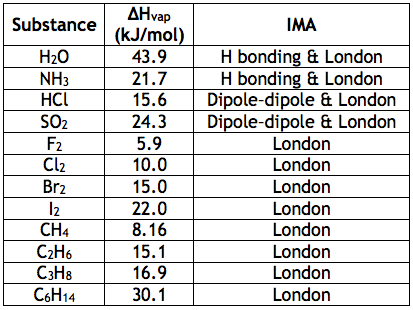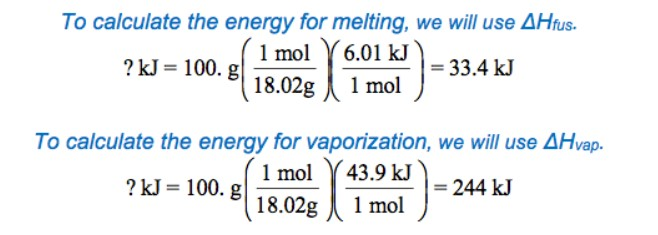# Calculating Energy Change – Phase Change

Hopefully you will remember from the unit on liquids and solids that when heat energy is supplied to a solid, the temperature of the solid climbs steadily until the melting point is reached. Once the melting point is reached, even though we are still supplying heat energy to the system, the temperature remains constant while the phase changes from solid to liquid. After the phase change is complete, the temperature starts to climb again. We drew this on a heating curve like the one shown below.Energy must be transferred to a system to cause it to melt or boil. The energy of the system therefore increases as the system undergoes a solid-liquid (or liquid-gas) phase change. Likewise, a system gives off energy when it freezes (or condenses). The energy of the system decreases as the system undergoes a liquid-solid (or gas-liquid) phase change.

The heat energy which a solid absorbs when it melts is called heat of fusion. (The word fusion means the same thing as melting. This sounds backwards, doesn’t it? Just think of fusion as the word to describe the liquid/solid phase change.   It might be easier to remember fusion if you think about ice forming from liquid water.) Heat of fusion is also called enthalpy of fusion. The term enthalpy will be defined on another page. Heat of fusion has the symbol ΔH fus. (H is the symbol for enthalpy.) ΔH fus is most often expressed in kJ or in kJ/mol. When it is expressed in kJ/mol, it is referred to as molar heat of fusion.

The amount of energy released when the same liquid changes into a solid is the same magnitude as ΔH fus, but it has the opposite sign, indicating that energy was released.

HEAT OF FUSION

+ΔH fus = energy absorbed during melting

-ΔH fus = energy released during freezing

The amount of energy needed to change a liquid into a gas (boiling) is called heat of vaporization. The amount of energy needed to vaporize one mole of a pure substance is called molar heat of vaporization, and the energy released in condensation has an equal magnitude.

HEAT OF VAPORIZATION

+ΔH vap = energy absorbed during boiling

-ΔH vap = energy released during condensation

## Relating Heat of Vaporization to IMAHeat energy is absorbed when a liquid boils because molecules which are held together by mutual attraction in the liquid are separated from each other as the gas is formed. Such a separation requires energy. The energy needed to separate the molecules in a liquid depends on the magnitude of the intermolecular forces (IMA)

Notice in the chart that the heats of vaporization of water and ammonia are very large, which is just what we would expect for hydrogen-bonded substances. By comparison, CH4, a nonpolar substance composed of atoms of similar size, has a very small heat of vaporization. Note also that polar substances such as HCl and SO2 have fairly large heats of vaporization compared to nonpolar substances. For example, compare HCl to Cl2. Even though Cl2 contains two relatively large atoms, and therefore would be expected to have larger London forces than HCl, the latter substance has the larger ∆Hvap. This must be due to dipole-dipole attractions between polar HCl molecules—attractions that are absent in nonpolar Cl2. We can thus expect liquids with strong intermolecular forces to have larger heats of vaporization.

Similar trends can be seen in heats of fusion as well. Solids, like ice, that have strong intermolecular force s have much higher molar heats of fusion than those like CH4 with weak ones.

## Calculations with Heat of Fusion and Heat of Vaporization

EQUATIONS TO CALCULATE HEAT

qlost = qgained

You will sometimes see this equation written with a negative sign, like this: qlost = -qgained

If you calculate ΔT correctly, however, paying attention to the signs, this negative sign will already be present in your equation.

Example

Compare the heat energy required to vaporize 100.0 g of water to the heat energy required to melt 100.0 g water.

Whenever you see the words vaporize, melt, and other words that indicated a phase change, you may need to use heat of fusion or heat or vaporization to solve that problem.  So, be on the lookout for those clues.

You can use both ΔH vap and ΔH fus as conversion facts or you can look at those units and write a formula.It takes more energy to change water from a liquid to a gas than it does to change the same amount of water from a solid to a liquid.

The heat of vaporization of a substance is always higher than its heat of fusion.

PRACTICE:

53.1 g H2O exists as a liquid at 0°C. How many kJ must be removed to turn the water into a solid at 0°C?

Hint – You will need to look up a constant to solve!

You have to look up the heat of fusion for water to solve this problem.
q = (6.02 kJ / mol) (53.1 g / 18.0 g/mol)

Now, watch this video showing you how to combine energy associated with phase changes with the energy for heating and cooling you learned about on the previous page.

For another look at calculating energy changes associated with a heating curve, watch this video from Khan Academy.

source